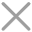﻿ Intro.js – 为您的网站提供更好的用户指引_dowebok

# Intro.js – 为您的网站提供更好的用户指引## 使用方法

### 引入文件

```<link rel="stylesheet" href="css/introjs.css">
<script src="js/intro.js"></script>```

```<!--[if lte IE 8]>
<!-- <![endif]-->```

### HTML

```<div><input class="btn" type="button" value="开始"></div>
<div data-step="2" data-intro="第二步，你好！">你好，这是第二步。</div>
<div data-step="1" data-intro="第一步，欢迎！">欢迎，这是第一步。</div>
<div data-step="3" data-intro="第三步，很好！">很好，这是第三步。</div>```

data-step 是步骤，data-intro 是介绍。

### JavaScript

```\$(function(){
var \$btn = \$('.btn');
\$btn.on('click', function(){
introJs().setOptions({
nextLabel: '下一步 &rarr;',
prevLabel: '&larr; 上一步',
skipLabel: '退出'
}).start();
});
});```

Intro.js 的 github 地址是：https://github.com/usablica/intro.js，里面有更详细的介绍。## 相关文章

##### 下载：Intro.js – 为您的网站提供更好的用户指引